Data Editing and Correction

There are several modules that enable direct or indirect editing of the SPM data. In principal, most of the data processing modules change the data in one way or another. However, in this section we would like to describe the modules and tools that are specifically designed to correct local defects in an image. The functions below remove bad data from an image, and then fill it in using an interpolation algorithm.

Align Rows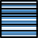Profiles taken in the fast scanning axis (usually x-axis) can be mutually shifted by some amount or have slightly different slopes. The basic line correction function Data ProcessCorrect DataAlign Rows deals with this type of discrepancy using several different correction algorithms:

Median

A basic correction method, based on finding a representative height of each scan line and subtracting it, thus moving the lines to the same height. Here the line median is used as the representative height.

Modus

This method differs from Median only in the quantity used: modus of the height distribution. Of course, the modus is only estimated because only a finite set of heights is avaiable.

Polynomial

The Polynomial method fits a polynomial of given degree and subtracts if from the line. For polynomial degree of 0 the mean value of each row is subtracted. Degree 1 means removal of linear slopes, degree 2 bow removal, etc.

Median difference

In contrast to shifting a representative height for each line, Median difference shifts the lines so that the median of height differences (between vertical neighbour pixels) becomes zero. Therefore it better preserves large features while it is more sensitive to completely bogus lines.

Matching

This algorithm is somewhat experimental but it may be useful sometimes. It minimizes a certain line difference function that gives more weight to flat areas and less weight to areas with large slopes.

Trimmed mean

Trimmed mean lies between the standard mean value and median, depending on how large fraction of lowest and highest values are trimmed. For no trimming (0) this method is equivalent to mean value subtraction, i.e. Polynomial with degree 0, for maximum possible trimming (0.5) it is equivalent to Median.

Trimmed mean of differences

This method similarly offers a continous transition between Median difference and mean value subtraction. It makes zero the trimmed means of height differences (between vertical neighbour pixels). For the maximum possible trimming (0.5) it is equivalent to Median difference. Since the mean difference is the same as the difference of mean values (unlike for medians), for no trimming (0) it is again equivalent to Polynomial with degree 0.

Similarly as in the two-dimensional polynomial levelling, the background, i.e. values subtracted from individual rows can be extracted to another image. Or plotted in a graph since the value is the same for the entire row.

The line correction function support masking, allowing the exclusion of large features that could distract the corection algorithms. The masking options are offered only if a mask is present though. Note the Path level tools described below offers a different method of choosing the image parts important for alignment. It can be more convenient in some cases.

Tip

Use Ctrl-F (Repeat Last) to run the line correction with the same settings on several images without going through the dialogue.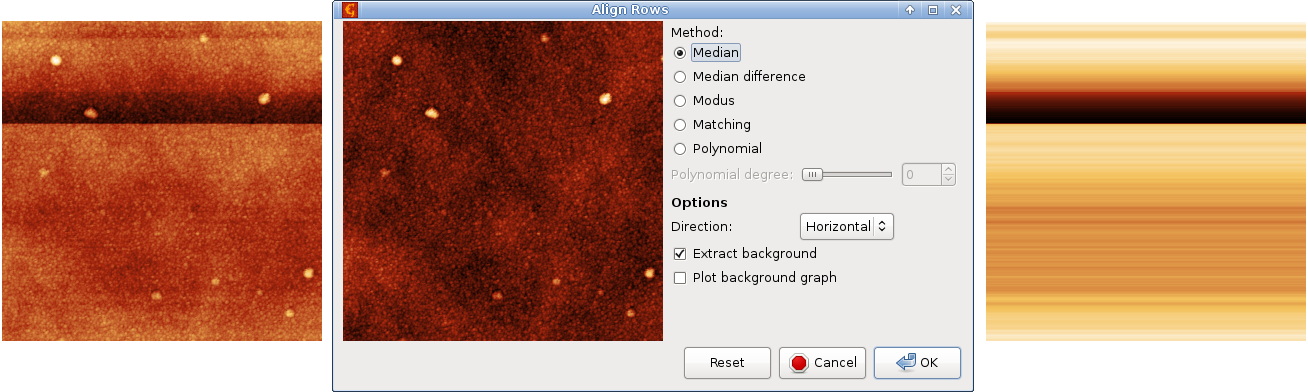Line correction example: an image with defects (left), the row alignment dialogue previewing the median correction (centre), and extracted row background (right). Note the false colour scales are not the same in the three images.

Step Line Correction

Function Step Line Correction attempts to deal with shifts that may occur in the middle of a scan line. It tries to identify misaligned segments within the rows and correct the height of each such segment individually. Therefore it is often able to correct data with discontinuities in the middle of a row. This function is somewhat experimental and the exact way it works can be subject to futher changes.

Mark Inverted Rows

Function Mark Inverted Rows finds scan lines with vertically inverted features and marks them with a mask. Line inversion is an artefact which occasionally occurs for instance in Magnetic Force Microscopy. Since the line is generally only inverted very approximately, value inversion would be a poor correction and one should usually use Laplace's interpolation for correction.

Remove Spots Tool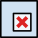The Remove Spots tool can be used for removing very small parts of the image that are considered a scanning error, dust particle or anything else that should not be present in the data. Note that doing so can dramatically alter the resulting statistical parameters of the surface, so be sure not to remove things that are really present on the surface.

While using this tool you can pick up position of the spot to magnify its neighbourhood in the tool window. Then, in the tool window, select a rectangle or ellipse (depending on chosen Shape) around the area that should be removed. You can then select one of several interpolation methods for creating data in place of the former spot:

• Hyperbolic flatten uses information from outer boundary pixels of the selected area to interpolate the information inside area.
• Laplace solver solves Laplace's equation to calculate data inside area; the outer boundary is treated as virtual source.
• Pseudo-Laplace is a faster approximation of true Laplace equation solution. For small areas one can always use the true solver.
• Fractal correction uses whole image to determine fractal dimension. Then tries to create randomly rough data that have the same fractal dimension and put them into the area.
• Fractal-Laplace blend is a weighted average of fractal interpolation and Laplace solver. The interior is given by fractal interpolation, but close to region edges the Laplace interpolation is mixed in to provide a smoother continuation to the exterior.

Clicking will execute the selected algorithm.

Note

Spot removal will only work for regions of size 80 × 80 pixels or smaller. To remove larger regions, create a mask using the Mask Editor tool, then use Data ProcessCorrect DataRemove Data Under Mask.

Remove Grains Tool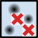The simple Remove Grains tool removes manually selected connected parts of mask or interpolates the data under them, or possibly both. The part of mask to remove is selected by clicking on it with left mouse button.

The data available interpolation methods are a subset of those offered by Remove Spots tool, in particular Laplace's equation solution, Fractal correction and their blend.

Remove Scars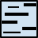Data ProcessCorrect DataRemove Scars

Scars (or stripes, strokes) are parts of the image that are corrupted by a very common scanning error: local fault of the closed loop. Line defects are usually parallel to the fast scanning axis in the image. This function will automatically find and remove these scars, using neighbourhood lines to fill-in the gaps. The method is run with the last settings used in Mark Scars.

Mark Scars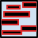Data ProcessCorrect DataMark Scars

Similarly, the `Mark Scars` module can create a mask of the points treated as scars. Unlike Remove Scars which directly interpolates the located defects, this module lets you interactively set several parameters which can fine-tune the scar selection process:

• Maximum width – only scars that are as thin or thinner than this value (in pixels) will be marked.
• Minimum length – only scars that are as long or longer than this value (in pixels) will be marked.
• Hard threshold – the minimum difference of the value from the neighbouring upper and lower lines to be considered a defect. The units are relative to image RMS.
• Soft threshold – values differing at least this much do not form defects themselves, but they are attached to defects obtained from the hard threshold if they touch one.
• Positive, Negative, Both – the type of defects to remove. Positive means defects with outlying values above the normal values (peaks), negative means defects with outlying values below the normal values (holes).

After clicking the new scar mask will be applied to the image. Other modules or tools can then be run to edit this data.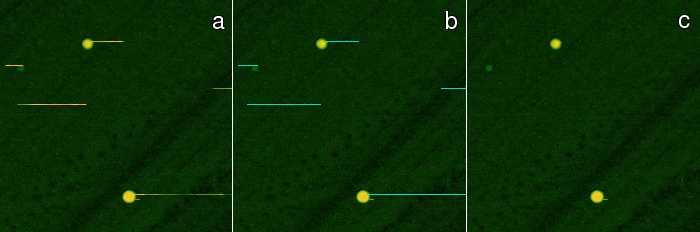Scars marking and removal example: (a) original data with defects, (b) data with marked deffects, (c) corrected data.

Remove Data Under Mask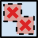Data ProcessCorrect DataRemove Data Under Mask

This function substitutes the data under the mask by the solution of solving the Laplace's equation. The data values around the masked areas define the boundary conditions. The solution is calculated iteratively and it can take some time to converge.

Fractal Correction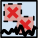Data ProcessCorrect DataFractal Correction

The Fractal Correction module, like the Remove Data Under Mask module, replaces data under the mask. However, it uses a different algorithm to come up with the new data: The fractal dimension of the whole image is first computed, and then the areas under the mask are substituted by a randomly rough surface having the same fractal dimension. The root mean square value of the height irregularities (roughness) is not changed by using this module.

Note

This calculation can take some time, so please be patient.

Warning

Running this module on data that do not have fractal properties can cause really unrealistic results and is strictly not recommended.

Mask of Outliers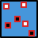This module creates mask of areas in the data that not pass the criterion. All the values above and below this confidence interval are marked in mask and can be edited or processed by other modules afterwards, for instance Remove Data Under Mask. This outlier marking method is useful for global outliers with values very far from the rest of the data.

Mask of Disconnected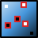Local outliers are values that stick out from their neighbourhood. Function Mask of Disconnected marks data that do not seem to belong in the distribution of surrounding values.

The outlier type to mark can be selected as Positive, Negative or Both for values much larger than their neighbours, much smaller and both types simultaneously, respectively. Note that selecting Both can mark different areas than if positive and negative outliers were marked separately and the results combined.

The marking proceeds by subtracting a local background from the image and then marking global outliers in the resulting flattened image. Specifically, the local background is obtained by an opening (minimum), closing (maximum) or a median filter of given radius. The radius of the filter is controlled with Defect radius. It determines the maximum size of defect that can be found and marked. However, it is often useful to use larger radius than the actual maximum defect size.

Defect marking sensitivity is controlled with option Threshold. Smaller values mean more conservative marking, i.e. less values marked as outliers. Larger value means more values marked as outliers.

Path Leveling Tool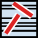The Path Leveling tool can be used to correct the heights in an arbitrary subset of rows in complicated images.

First, one selects a number of straight lines on the data. The intersections of these lines with the rows then form a set of points in each row that is used for leveling. The rows are moved up or down to minimize the difference between the heights of the points of adjacent rows. Rows that are not intersected by any line are not moved (relatively to neighbouring rows).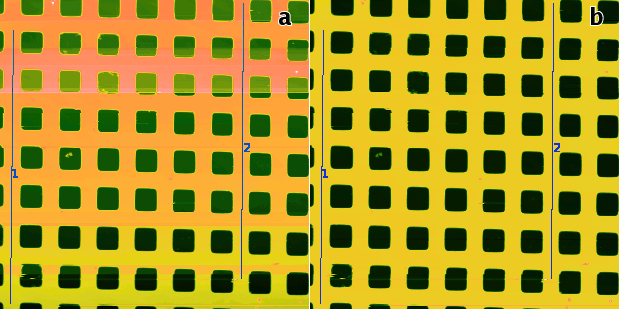Path Level example: (a) uncorrected data with steps that the automated method may fail to correct, two suitable leveling lines are selected; (b) the result of Path Level application with line width 5.

Unrotate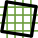Data ProcessCorrect DataUnrotate

Unrotate can automatically make principal directions in an image parallel with horizontal and/or vertical image edges. For that to work, the data need to have some principal directions, therefore it is most useful for scans of artifical and possibly crystalline structures.

The rotation necessary to straighten the image – displayed as Correction – is calculated from peaks in angular slope distribution assuming a prevalent type of structure, or symmetry. The symmetry can be estimated automatically too, but it is possible to select a particular symmetry type manually and let the module calculate only corresponding rotation correction. Note if you assume a structure type that does not match the actual structure, the calculated rotation is rarely meaningful.

It is recommended to level (or facet-level) the data first as overall slope can skew the calculated rotations.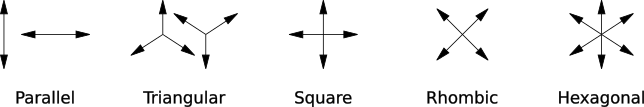Orientations of prevalent directions corresponding to Unrotate symmetry types.

The assumed structure type can be set with Assume selector. Following choices are possible:

Detected

Automatically detected symmetry type, displayed above as Detected.

Parallel

Parallel lines, one prevalent direction.

Triangular

Triangular symmetry, three prevalent directions (unilateral) by 120 degrees.

Square

Square symmetry, two prevalent directions oriented approximately along image sides.

Rhombic

Rhombic symmetry, two prevalent directions oriented approximately along diagonals. The only difference from Square is the preferred diagonal orientation (as opposed to parallel with sides).

Hexagonal

Hexagonal symmetry, three prevalent directions (bilateral) by 120 degrees.